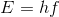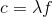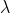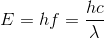# College Physics : Fundamental Concepts

## Example Questions

### Example Question #1 : Waves

When a ray of light is reflected off of the surface of another medium, some of the energy from the light is transferred into this medium. As a result, which of the following is true about the reflected light ray?

It has a lower frequency and a higher wavelength

It has a higher frequency and a higher wavelength

It has a lower frequency and a lower wavelength

It has a higher frequency and a lower wavelength

It has a lower frequency and a higher wavelength

Explanation:

For this question, we're told that light is reflected off of the surface of another medium. As a result, the light loses some of its energy, which is transferred into the medium where it reflected.

Because the light has lost some of its energy, we need to determine how the wavelength and frequency of the wave will be affected. To do this, we can recall the equation for the energy of a wave.Whereis Planck's constant andrepresents the frequency. We can see that when energy is decreased, the frequency also decreases. Thus, we can eliminate two of the answer choices.

To see how the wavelength changes, it's important to recall the relationship that wavelength and frequency have with each other.Whereis the speed of light andis the wavelength. Since the light is still traveling in the same medium, its speed will not change. Thus, as the frequency of the wave decreases, the wavelength has to increase. We can alternatively show how all of these variables are related as follows.So, in summary, when the energy of light decreases, the frequency will decrease and the wavelength will increase.

### All College Physics Resources